### Hyperelastic material - HyperMat

This material model can describe elastic behavior at large strains. A hyperelastic model postulates the existence of free energy potential. Existence of the potential implies reversibility of deformations and no energy dissipation during loading process. Here we use the free energy function introduced in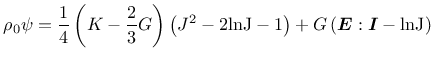(9)

where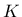is the bulk modulus,is the shear modulus,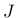is the Jacobian (determinant of the deformation gradient, corresponding to the ratio of the current and initial volume) and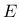is the Green-Lagrange strain. Then stress-strain law can be derived from (9) as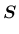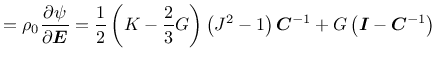(10)

where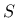is the second Piola-Kirchhoff stress,is the Green-Lagrange strain andis the right Cauchy-Green tensor. The model description and parameters are summarized in Tab. 4.

Table 4: Hyperelastic material - summary.
 Description Hyperelastic material Record Format HyperMat (in) # d(rn) # K(rn) # G(rn) # Parameters - material number - d material density - K bulk modulus - G shear modulus Supported modes 3dMat

Borek Patzak
2019-03-19